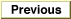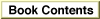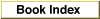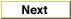# Legacy DocumentImportant: The information in this document is obsolete and should not be used for new development.

Inside Macintosh: QuickDraw GX Environment and Utilities /
Chapter 8 - QuickDraw GX Mathematics / QuickDraw GX Mathematics Reference
Mathematical Functions / Fixed-Point Operations

### Magnitude

You can use the `Magnitude` function to obtain the magnitude of a vector, the length of a line, or the distance between two points.

```unsigned long Magnitude (long deltaX, long deltaY);
```
`deltaX`
The difference in the x-coordinates of the vector's end points.
`deltaY`
The difference in the y-coordinates of the vector's end points.
function result
The magnitude of the vector.
##### DESCRIPTION
The `Magnitude` function returns (`deltaX2` + `deltaY2`)1/2, the Euclidean distance between two points whose x-coordinates are separated by `deltaX` and whose y-coordinates are separated by `deltaY`.

The fixed-point format of the result is the same as the fixed-point format for both of the parameters. Make sure that the two parameters use the same format.© Apple Computer, Inc.
7 JUL 1996